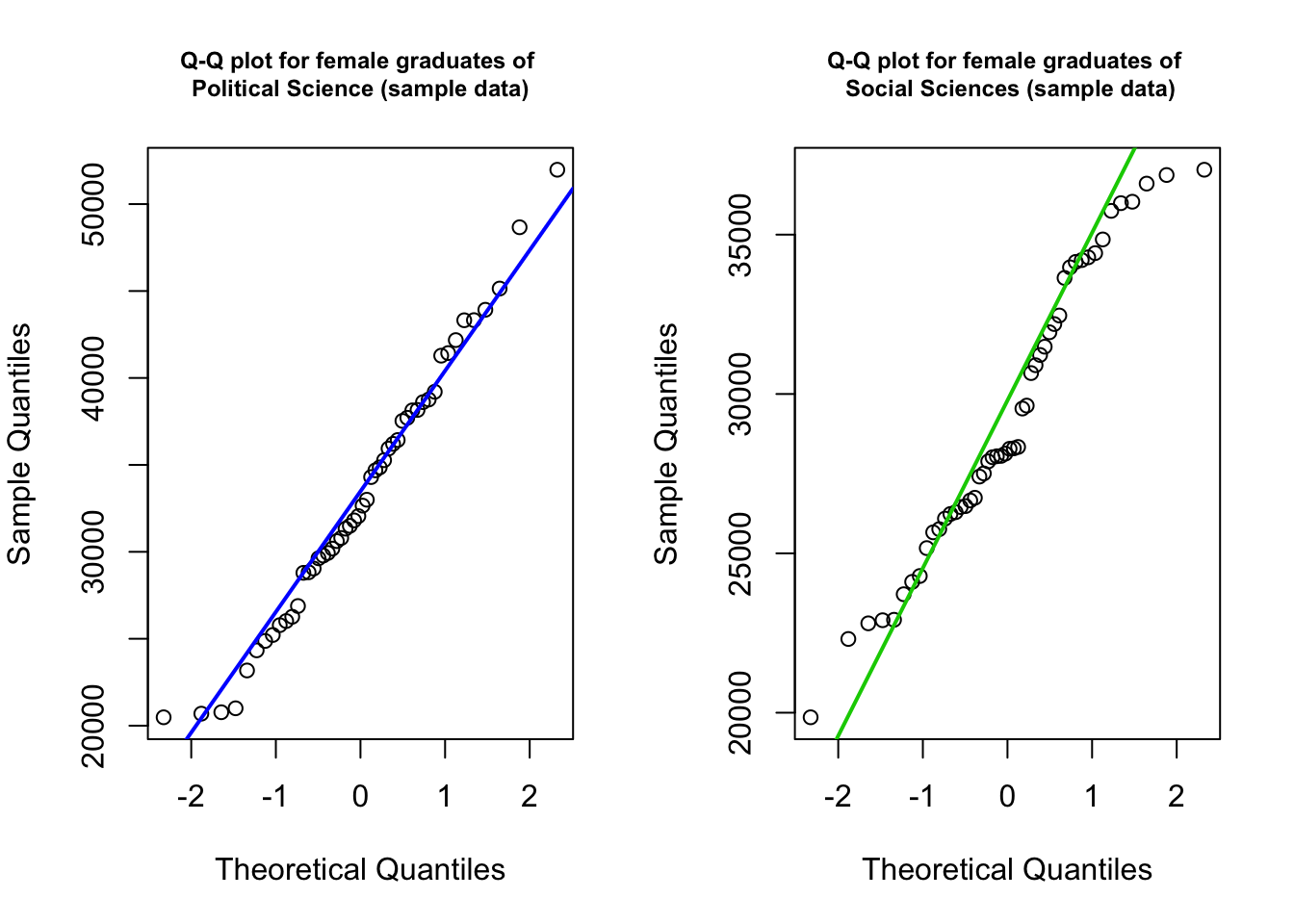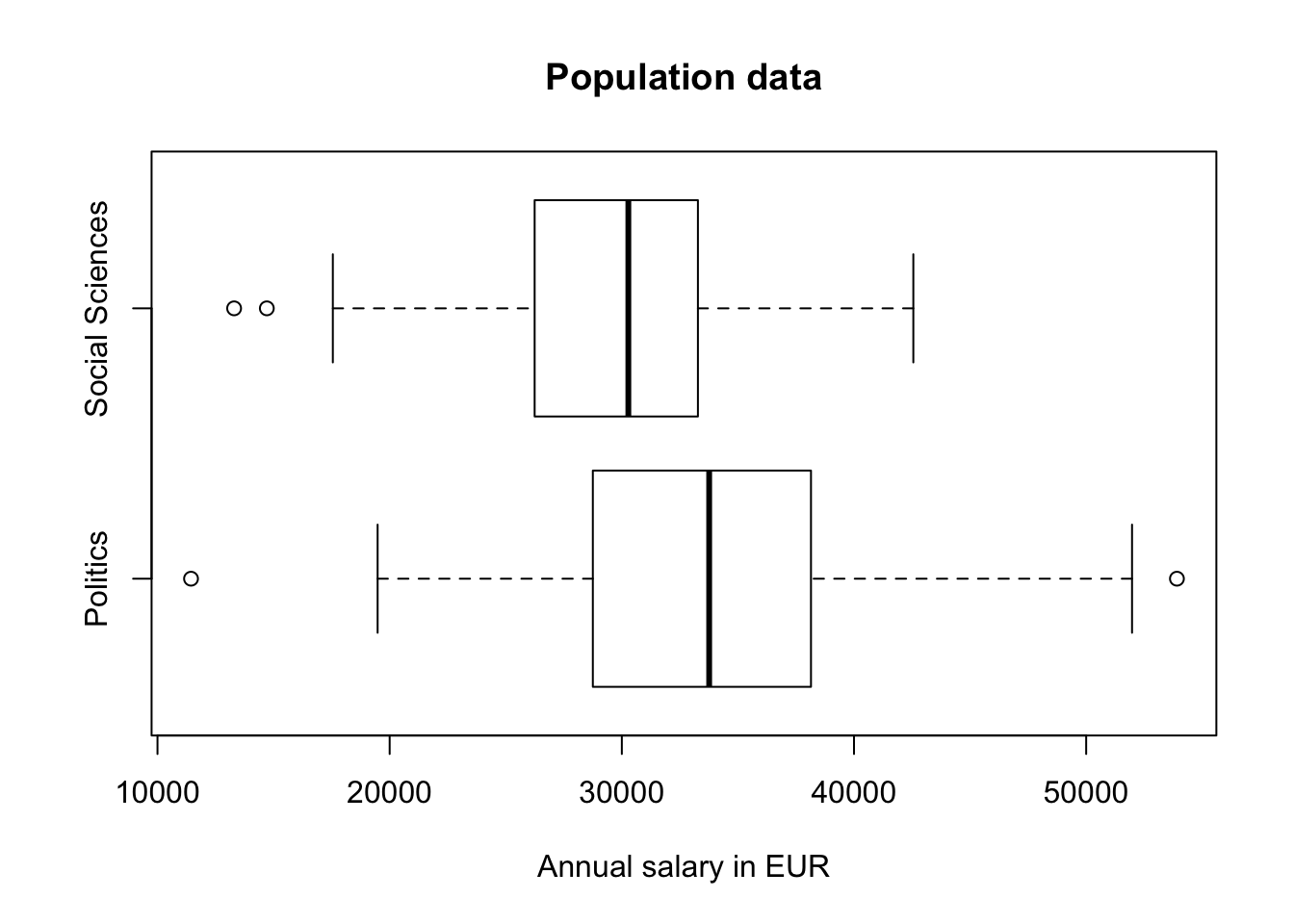In cases we want to test for two population means and the standard deviations are different between the two populations, the so-called non-pooled t-test or Welch’s t-test is applied.

The non-pooled t-test is very similar to the pooled t-test, except for the test statistic $$t$$ and for the calculation of the degrees of freedom $$(df)$$. The test statistic does not invoke $$s_p$$, the pooled standard deviation, and is written as

$t = \frac{(\bar x_1 - \bar x_2)}{ \sqrt{\frac{s_1^2}{n_1} + \frac{s_2^2}{n_2}}}\text{.}$

The denominator of the equation from above is the estimator of the standard deviation of $$\bar x_1 - \bar x_2$$, given by

$s_{\bar x_1 - \bar x_2} = \sqrt{\frac{s^2_1}{n_1} + \frac{s^2_2}{n_2}}\text{.}$

The test statistics $$t$$ has a t-distribution and the degrees of freedom $$(df)$$ are given by

$df=\frac{\left(\frac{s_1^2}{n_1}+\frac{s_1^2}{n_2}\right)^2}{\frac{\left(\frac{s_1^2}{n_1}\right)^2}{n_1-1}+\frac{\left(\frac{s_2^2}{n_2}\right)^2}{n_2-1}}\text{.}$ Round down the degrees of freedom to the nearest integer if you use look-up tables.

The non-pooled t-test is robust to moderate violations of normal population assumption, but it is less robust regarding outliers (Weiss 2010).

#### Interval Estimation of $$\mu_1 - \mu_2$$

The $$100(1-\alpha)$$% confidence interval for $$\mu_1 - \mu_2$$ is

$(\bar x_1 - \bar x_2) \pm t^* \times \sqrt{\frac{s^2_1}{n_1} + \frac{s^2_2}{n_2}}$

where the value of t is obtained from the t-distribution for the given confidence level. The degrees of freedom $$(df)$$ and the are obtained from the equation above.

#### The Non-Pooled t-Test: An Example

In order to get some hands-on experience we apply the non-pooled t-test in an exercise. Therefore we load the students data set. You may download the students.csv file here. Import the data set and assign a proper name to it.

students <- read.csv("https://userpage.fu-berlin.de/soga/200/2010_data_sets/students.csv")

The students data set consists of 8239 rows, each of them representing a particular student, and 16 columns, each of them corresponding to a variable/feature related to that particular student. These self-explaining variables are: stud.id, name, gender, age, height, weight, religion, nc.score, semester, major, minor, score1, score2, online.tutorial, graduated, salary.

In order to showcase the non-pooled t-test we examine the mean annual salary (in Euro) of female graduates with respect to their major study subject. The first population consists of female students with their major in Political Science and the second population of female students with their major in Social Sciences. We want to test, whether there is a difference in the mean salary of these two groups?

#### Data preparation

• We subset the data set based on the variables gender and graduated.
• Then we split the subset into graduates of Political Science and Social Sciences (variable major), respectively.
• Then we sample from each group 50 students and extract the variable of interest, the mean annual salary (in Euro), which is stored in the column salary. We assign those two vectors to the variables PS and SS.
female.graduates <- subset(students, graduated==1 & gender=='Female')

n <- 50
PS <- sample(subset_PS$salary, n) SS <- sample(subset_SS$salary, n)

Further we check if the data is normally distributed by plotting a Q-Q plot. In R we apply the qqnorm() and the qqline() functions for plotting Q-Q plots.

par(mfrow = c(1,2), mar = c(5,4,4,2))

qqnorm(PS, main = 'Q-Q plot for female graduates of \nPolitical Science (sample data)', cex.main=0.75)
qqline(PS, col = 4, lwd=2)

qqnorm(SS, main = 'Q-Q plot for female graduates of \n Social Sciences (sample data)', cex.main=0.75)
qqline(SS, col = 3, lwd=2)We see that the data of both samples falls roughly on a straight line.

Let us assume that the data of the students data set is a good approximation for the population. Then, we may check visually if the standard deviations of the two populations actually differ from each another by plotting a a box plot.

boxplot(subset_PS$salary, subset_SS$salary,
horizontal = TRUE,
names = c('Politics', 'Social Sciences'),
xlab = 'Annual salary in EUR',
main = "Population data")Based on the graphical evaluation approach we conclude that the data is roughly normally distributed and that the standard deviations differ from each another.

#### Hypothesis testing

Recall the research question. Do the data provide sufficient evidence to conclude that the mean annual salary of female graduates with a major in Political Science differs from the mean annual salary of female graduates with a major in Social Sciences?

In order to conduct the non-pooled t-test we follow the step-wise implementation procedure for hypothesis testing.

Step 1: State the null hypothesis $$H_0$$ and alternative hypothesis $$H_A$$

The null hypothesis states that the average annual salary of female graduates with a major in Political Science ($$\mu_1$$) is equal to the average annual salary of female graduates with a major in Social Sciences ($$\mu_2$$).

$H_0: \quad \mu_1 = \mu_2$

Alternative hypothesis $H_A: \quad \mu_1 \ne \mu_2$ This formulation results in a two-sided hypothesis test.

Step 2: Decide on the significance level, $$\alpha$$

$\alpha = 0.05$

alpha <- 0.05

Step 3 and 4: Compute the value of the test statistic and the p-value.

For illustration purposes we manually compute the test statistic in R. Recall the equations for the test statistic from above.

$t = \frac{(\bar x_1 - \bar x_2)}{ \sqrt{\frac{s_1^2}{n_1} + \frac{s_2^2}{n_2}}}$

# Compute the value of the test statistic
n1 <-length(PS)
n2 <- length(SS)
s1 <- sd(PS)
s2 <- sd(SS)
x1.bar <- mean(PS)
x2.bar <- mean(SS)

t <- (x1.bar-x2.bar)/(sqrt(s1^2/n1+s2^2/n2))
t
##  3.252054

The numerical value of the test statistic is 3.2520539.

In order to calculate the p-value we apply the pt() function. Recall how to calculate the degrees of freedom.

$df=\frac{\left(\frac{s_1^2}{n_1}+\frac{s_1^2}{n_2}\right)^2}{\frac{\left(\frac{s_1^2}{n_1}\right)^2}{n_1-1}+\frac{\left(\frac{s_2^2}{n_2}\right)^2}{n_2-1}}\text{,}$

# Compute df
df.numerator <- (s1^2/n1 + s2^2/n2)^2
df.denominator <- (s1^2/n1)^2/(n1-1) + (s2^2/n2)^2/(n2-1)
df = df.numerator/df.denominator
df
##  79.94333
# Compute the p-value
# recall we are applying a two-sided test
upper <- pt(abs(t), df = df, lower.tail = FALSE)
lower <- pt(-abs(t), df = df, lower.tail = TRUE)
p <- upper + lower
p
##  0.001678878

Step 5: If $$p \le \alpha$$, reject $$H_0$$; otherwise, do not reject $$H_0$$.

p <= alpha
##  TRUE

The p-value is less than the specified significance level of 0.05; we reject $$H_0$$. The test results are statistically significant at the 5% level and provide very strong evidence against the null hypothesis.

Step 6: Interpret the result of the hypothesis test.

$$p = 0.0016789$$. At the 5% significance level, the data provides very strong evidence to conclude that the average annual salary of female graduates of Politcal Science differs from the average annual salary of female graduates of Social Sciences.

#### Hypothesis testing in R

We just completed a non-pooled t-test in R manually. Now we make use of the full power of the R machinery to obtain the same result as above by just one line of code!

In order to conduct a non-pooled t-test in R we apply the t.test() function. We provide two vectors as data input, and further, we set var.equal = FALSE, in order to explicitly state, that we apply the non-pooled version of the t-test. We do not need to set the alternative argument, as the default value corresponds to our alternative hypothesis $$H_A: \; \mu_1 \ne \mu_2$$.

t.test(x = PS, y = SS, var.equal = FALSE)
##
##  Welch Two Sample t-test
##
## data:  PS and SS
## t = 3.2521, df = 79.943, p-value = 0.001679
## alternative hypothesis: true difference in means is not equal to 0
## 95 percent confidence interval:
##  1553.956 6455.035
## sample estimates:
## mean of x mean of y
##  33249.20  29244.71

Super powerful! Compare the output of the t.test() function with our result from above. They match perfectly! Again, we may conclude that at the 5% significance level, the data provides very strong evidence to conclude that the average annual salary of female graduates of Politcal Science differs from the average annual salary of female graduates of Social Sciences.